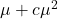# Variance function in semi-parametric analysis of count data

The purpose of this paper is to determine an appropriate variance function
(mean-variance relationship) which can be used in the semi-parametric analy-
sis of over-dispersed count data (for example, for analysis of count data by ex-
tended quasi-likelihood and double extended quasi-likelihood). We use hypothesis
testing approach through a broader class of models and data analytic approach.
The models considered are the three parameter negative binomial distribution
and the extended quasi-likelihood. Wide analysis involving tests, data analysis
and simulations indicate that the three parameter generalized negative binomial
distribution does not improve in t to count data over the simpler negative bi-
nomial distribution. Further data analysis and simulations using the extended
quasi-likelihood indicate that the negative binomial variance functionis
preferable over a simpler variance functionfor data with small mean and
small over-dispersion. Otherwiseis a preferable variance function over the
negative binomial variance function.

44n1_9